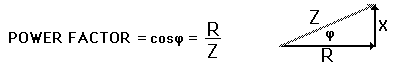Home » Current Electricity » Power factor in AC circuits

# Power factor in AC circuits

“what is meant by power factor?”

Ans:

In AC circuits, Power factor is defined as the ratio of true power to apparent power.$Power Factor =\frac{True Power}{Apparent Power}$

If φ is the phase difference between E and I, then

True Power = EvIv cos φ and the Apparent Power is EvIv

Therefore,This site uses Akismet to reduce spam. Learn how your comment data is processed.

### Hits so far @ AskPhysics

• 2,274,296 hits